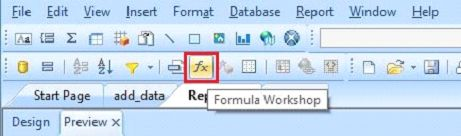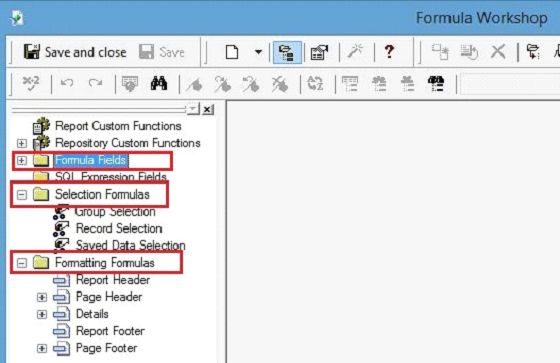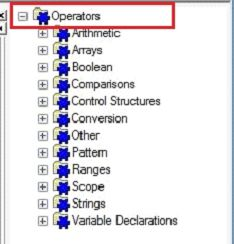# Formula Workshop

In Crystal Report, we use Formula Workshop to create different types of formulas. It calculates a large amount of data at one time. Now, we see the work of 'Formula Workshop'.

We can open Formula Workshop by clicking on this tab, which is shown in the image, given below,Here, we see some main parts of Formula Workshop - Formula Fields, Selection Formula, Formatting Formula etc.Formulas Field

It holds the formula name in Crystal Report.

Result Objects

It holds the result, which is used in the report.

Formula Text
It is used to create or edit the formulas.

Workshop Buttons
It is used to filter, sort, delete the formulas etc.

Operators
Operators are used to apply the conditions between values. Example - arithmetic, string etc.Functions
It holds the inbuilt functions, which can be used in the report. Example - add, date time, date ranges, financial etc.Summary

Thus, we learnt, Formula Workshop is used to create different type of formula in Crystal Report.
Next » Create And Modify Formulas